# Equations

Equation: a mathematical sentence that contains two expressions and is separated by an equal sign (both sides of the equation have the same value).

Example:         4 + 3 = 7,         9x – 4 = 5,            2y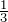= y

To solve an equation we find a particular value for the variable in the equation that makes the equation true (left side = right side).

Example:   For the equation   x + 4 = 5

only x = 1 can make it true, since   1 + 4 = 5         (Left side = Right side)

Solution of an equation: the value of the variable in the equation that makes the equation true.

Example:    For the equation   x + 4 = 5,     x = 1   is the solution.

Example 1.2.1

Indicate whether each of the given number is a solution to the given equation.

 1)      2 :      4x – 3 = 5 4 ∙ 2 – 3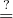5 5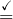5 Yes Replace x with 2.
 2)      15 :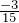y = -3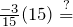-3 -3-3 Yes Replace y with 15.
 3)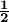:       8t = 3 8 (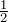)3 4 ≠ 3 No Cannot replace t with.

# Basic rules for solving one-step equations:

• Add, subtract, multiply or divide the same quantity to both sides of an equation to obtain a valid equation.
• Remember to always do the same thing to both sides of the equation (balance).

Properties for solving equations:

 Properties Equality Example
 Addition property of equality A = B       A + C = B + C Solve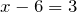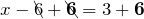x = 9 Subtraction property of equality A = B       A – C = B – C Solve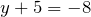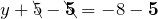y = -13 Multiplication property of equality A = B        A · C = B · C Solve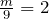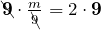m = 18 Division property of equality A = B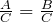(C ≠ 0) Solve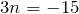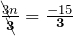n = –5

Example 1.2.2

Solve the following equations.

 1)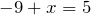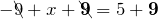Property of addition.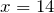Check: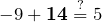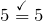Replace x with 14.

 2)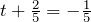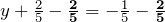Property of subtraction.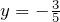3)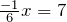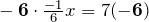Property of multiplication.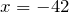4)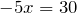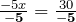Property of division.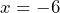Multi-step equation: an equation that requires more than one step to solve it.

Procedure for solving multi-step equations:

 Clear the fractions or decimals if necessary. Simplify and remove parentheses if necessary. Combine like terms on each side of the equation. Collect the variable terms on one side of the equation and the constants on the other side. Isolate the variable (to get the variable alone on one side of the equation). Check the solution with the original equation.

 Steps Example
 Solve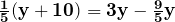Eliminate the denominators if the equation has fractions.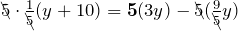Multiply each term by 5. Remove parentheses.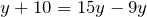Combine like terms.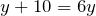Collect variable terms on one side and the constants on the other side.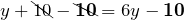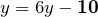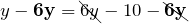Subtract 10 from both sides.   Subtract 6y from both sides. Isolate the variable.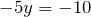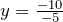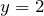Divide both sides by -5. Check with the original equation.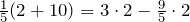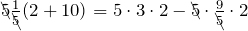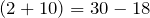Replace y with 2. Multiply each term by 5.   LS = RS  (correct)

Equations involving decimals: Multiply every term of both sides of the equation by a multiple of 10 (10, 100, 1000, etc.) to clear the decimals (based on the number with the largest number of decimal places in the equation).

 Steps Example
 Solve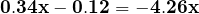Multiply each term by 100 to clear the decimal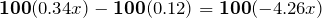The largest number of decimal place is two. Collect the variable terms on one side of the equation and the constants on the other side.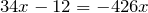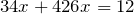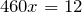Add 12 to both sides. Add 426x to both sides. Isolate the variable.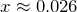Example 1.2.3

 Solve   0.4y + 0.08 = 0.016 The largest number of decimal place is three. 1000(0.4y) + 1000(0.08) = 1000(0.016) Multiply each term by 1000. 400y + 80 = 16 Combine like terms. 400y = -64 Divide both sides by 400. y = – 0.16

Equations involving fractions:

 Steps Example
 Solve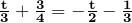Multiply each term by the lowest common denominator (LCD).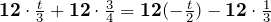Collect the variable terms on one side of the equation and the constants on the other side.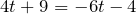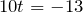Add 6t to both sides. Subtract 9 from both sides. Isolate the variable.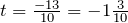Divide both sides by 10.

# Word Problems

Identifying keywords:

• When trying to figure out the correct operation ( + , – , × , ÷ , etc.) in a word problem it is important to pay attention to keywords (clues to what the problem is asking).
• Identifying keywords and pulling out relevant information that appear in the word problem are effective ways for solving mathematical word problems.

Key or clue words in word problems:

 Addition  (+) Subtraction (–) Multiplication (×) Division  (÷) Equals to  (=)
 add subtract times divided by equals sum (of) difference product quotient is plus take away multiplied by over was total (of) minus double split up are altogether less (than) twice fit into were increased by decreased by triple per amounts to gain (of) loss (of) of each totals combined (amount) left how much (total) goes into results in in all savings how many as much as the same as greater than withdraw out of gives complete reduced by ratio/rate yields together fewer (than) percent more (than) how much more share additional how long average

Example 1.2.4

1) Edward drove from Prince George to Williams Lake (235 km), then to Cache Creek (203 km) and finally to Vancouver (390 km). How many kilometres in total did Edward drive?

 235km + 203 km + 390 km = 828 km The key word: total (+)

2) Emma had $150 in her purse on Friday. She bought a pizza for$15, and a pair of shoes for $35. How much money does she have left? $150 – 15 – 35 = $100 The key word: left (–) 3) Lucy received$950 per month of rent from Mark for the months September to November. How much rent in total did she receive?

 $9503 =$2850 The key word: how much total (×)

4) Julia is going to buy a $7500 used car from her uncle. She promises to pay$500 per month. In how many months can she pay it off?

 $7500 ÷$500 = 15 months The key word: per (÷)

Steps for solving word problems:

 –      Organize the facts given from the problem (create a table or diagram if it will make the problem clearer) –      Identify and label the unknown quantity (let x = unknown). –      Convert words into mathematical symbols, and determine the operation – write an equation (looking for ‘key’ or ‘clue’ words). –      Estimate and solve the equation and find the solution(s). –      Check and state the answer. (Check the solution to the equation and check it back into the problem – is it logical?)

Example 1.2.5

William bought 5 pairs of socks for $4.35 each. The cashier charged him an additional$2.15 in sales tax. He left the store with a measly $5.15. How much money did William start with? • Organize the facts (make a table):  5 socks$4.35 each Sales tax $2.15 Money left$5.15
• Determine the unknown: How much did William start with?  (x = ?)
• Convert words into math symbols, and determine the operation (find keywords):
 The total cost without the sales tax: $4.35 × 5 With an additional$2.15 sales tax: ($4.35 × 5) +$2.15 Estimate and solve the unknown: x = [($4.35 × 5) +$2.15] + $5.15 • Estimate and solve the unknown:  Estimate: x = [($4 × 5) + $2] +$5 = $27 Actual solution: x = [($4.35 × 5) + $2.15] +$5.15 = $29.05 • Check: If William started with$29.05, and subtract 5 socks for $4.35 each and sales tax in$2.15 to see if it equals $5.15. $29.05 – [($4.35 × 5) +$2.15]$5.15$29.05 – $23.9$5.15 Correct!

More examples:

Example 1.2.6

James had 96 toys. He sold 13 on first day, 32 on second day, 21 on third day, 14 on fourth day and 7 on the last day. What percentage of the toys were not sold?

• Organize the facts:
 James had 96 toys The total number of toys sold 13 + 32 + 21 + 14 + 7 The toys not sold 96 – the total number of toys sold
 Determine the unknown: Let x = percentage of the toys were not sold The total number of toys sold: 13 + 32 + 21 + 14 + 7 = 87 The toys not sold: 96 – 87 = 9 Percentage of the toys were not sold: x =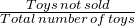=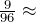0.094 = 9.4%

Example 1.2.7

The 60-litre gas tank in Robert’s car is 1/2 full. Kelowna is about 390 km from Vancouver and his car averages 7 litres per 100 km. Can Robert make his trip to Vancouver?

• Let x = litres of fuel are required to get to Vancouver.
• The 60-litre gas tank in Robert’s car is 1/2 full:
 60 L ×= 30 L Robert has 30 litres gas in his car.
• Robert’s car averages 7 litres per 100 km, and Vancouver is about 390 km from Kelowna.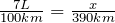Proportion: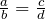(x)(100km) = (7 L) (390 km) Cross multiply and solve for x. x =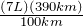= 27.3 L Robert needs 27.3 litres gas to get to Vancouver.
• 30L > 27.3L. Therefore, yes, Robert can make his trip.

# Practice questions

1. Solve the following equations:

a. x – 7 = 12

b. y +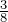=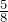c.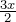=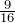d. 14t + 5 = 8

e. 7(x – 3) + 3x – 5 = 2(5 – 4x)

f.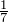(y + 12) = 4y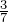y

g. 0.5t + 0.05 = 0.025

h.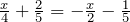2. Write an expression for each of the following:

a. Susan has \$375 in her checking account. If she makes a deposit of y dollars, how much in total will she have in her account?

b. Mark weighs 175 pounds. If he loses y pounds, how much will he weigh?

c. A piece of wire 45 metres long was cut in two pieces and one piece is w metres long. How long is the other piece?

d. Emily made 4 dozen muffins. If it cost her x dollars, what was her cost per dozen muffins? What was her cost per muffin?